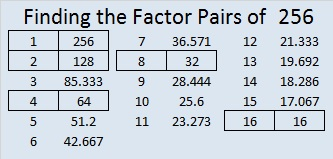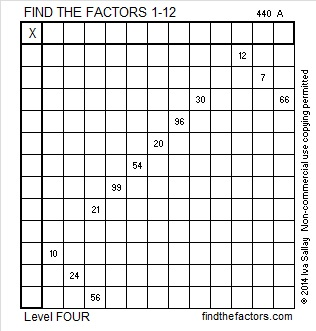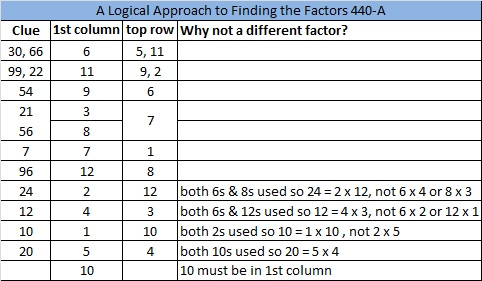# 256 and Level 4

• 256 is a composite number.
• Prime factorization: 256 = 2 x 2 x 2 x 2 x 2 x 2 x 2 x 2, which can be written 256 = 2⁸
• The exponent in the prime factorization is 8. Adding one we get (8 + 1) = 9. Therefore 256 has 9 factors.
• Factors of 256: 1, 2, 4, 8, 16, 32, 64, 128, 256
• Factor pairs: 256 = 1 x 256, 2 x 128, 4 x 64, 8 x 32, or 16 x 16
• 256 is a perfect square. √256 = 16.((2²)²)² = 256

1 + 3 + 5 + 7 + 9 + 11 + … + 29 + 31 = 256; that’s the sum of the first 16 odd numbers.

Because it is a power of 2, it is impossible to write 256 as the sum of consecutive whole numbers.

ALL of the factors of 256 are powers of 2: 2⁰=1, 2¹=2, 2²=4, 2³=8, 2⁴=16, 2⁵=32, 2⁶=64, 2⁷=128, 2⁸=256

NONE of the clues in this level 4 puzzle are powers of 2, but can you still solve it and figure out where the powers of 2 belong in the puzzle?Print the puzzles or type the factors on this excel file: 12 Factors 2014-10-06This site uses Akismet to reduce spam. Learn how your comment data is processed.